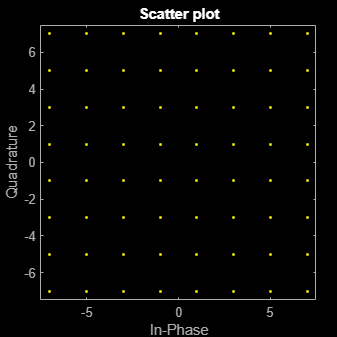# scatterplot

Generate scatter plot

## Syntax

``scatterplot(x)``
``scatterplot(x,n)``
``scatterplot(x,n,offset)``
``scatterplot(x,n,offset,plotstring)``
``scatterplot(x,n,offset,plotstring,scatfig)``
``scatfig = scatterplot(___)``

## Description

example

````scatterplot(x)` creates a scatter plot for signal `x`.```
````scatterplot(x,n)` specifies decimation factor `n`. The function plot every `n`th value of `x`, starting from its first value.```
````scatterplot(x,n,offset)` specifies the offset value. The function plots every `n`th value of `x`, starting from its (`offset` + 1)th value.```
````scatterplot(x,n,offset,plotstring)` specifies plot attributes for the scatter plot.```
````scatterplot(x,n,offset,plotstring,scatfig)` generates the scatter plot in the existing `Figure` object, `scatfig`. To plot multiple signals in the same figure, use `hold on`.```
````scatfig = scatterplot(___)` returns the `Figure` object of the scatter plot. Use `scatfig` to query or modify properties of the figure after it is created. You can specify any of the input argument combinations from the previous syntaxes.```

## Examples

collapse all

Create a 64-QAM signal in which each constellation point is used.

```d = (0:63)'; s = qammod(d,64);```

Display the scatter plot of the constellation.

`scatterplot(s)`## Input Arguments

collapse all

Input signal, specified as a vector or matrix.

The interpretation of `x` depends on its shape and complexity.

• If `x` is a real-valued two-column matrix, the function interprets the first column as in-phase components and the second column as quadrature components.

• If `x` is a complex-valued vector, the function interprets the real part as in-phase components and the imaginary part as quadrature components.

• If `x` is a real-valued vector, the function interprets it as a real signal.

Data Types: `double` | `single`
Complex Number Support: Yes

Decimation factor, specified as a positive integer. The function plots every `n`th value of input signal `x`, starting from its first value.

Data Types: `double`

Offset value, specified as a nonnegative integer. This offset value specifies the number of samples at the beginning of input `x` that the function skips before generating the scatter plot.

Data Types: `double`

Plot attributes, specified as a character vector or string scalar containing symbols.

This argument sets the plotting symbol, line type, and color for the scatter plot. The format and meaning of the symbols are the same as in the `plot` function. For example, the default value `'b.'` produces blue dots.

Data Types: `char` | `string`

Target scatterplot, specified as a `Figure` object for a previously generated `scatterplot`.

## Output Arguments

collapse all

Target scatterplot, returned as a `Figure` object. To modify properties of this object, see `Figure Properties`.

### Topics

Introduced before R2006a